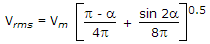# Electronics and Communication Engineering - Power Electronics

### Exercise :: Power Electronics - Section 1

21.

A two winding transformer is feeding a single phase half wave rectifier circuit. The load is purely resistive. The rms value of transformer secondary current is Is and rms value of load current in Irms. Then

 A. Is = Irms irrespective of the value of firing angle B. Is = 0.5 Irms irrespective of the value of firing angle C. Is = Irms if firing angle is less than 30° D. Is = Irms if firing angle is more than 30°

Explanation:.

22.

The terminals of a power BJT are called

 A. emitter, base, collector B. emitter, base, drain C. source, base, drain D. source, base, collector

Explanation:

In a BJT terminals are emitter, base and collector irrespective of rating.

23.

In single phase half wave regulator, the average current over one full cycle

 A. is always positive B. may be positive or negative C. may be negative D. is always negative

Explanation:

The input current during positive half cycle is less than that during negative half cycle.

24.

The dynamic equalising circuit consists of a series combination of capacitor C and resistor RC across each thyristor. This resistance RC (along with parallel connected diode)

 A. limits charging current B. limits discharging current C. limits both charging and discharging currents D. none of the above

Explanation:

Discharge current flows through Re .

25.

A single phase semiconverter is feeding a highly inductive load and has freewheeling diode across the load. The waveshapes of output voltage and output current

 A. are similar B. are not similar C. may be similar or dissimilar D. are similar only if firing angle is zero